# JP2019060699A - Displacement measuring device, system, and displacement measuring method - Google Patents

## Info

Publication number
JP2019060699A
JP2019060699A JP2017184995A JP2017184995A JP2019060699A JP 2019060699 A JP2019060699 A JP 2019060699A JP 2017184995 A JP2017184995 A JP 2017184995A JP 2017184995 A JP2017184995 A JP 2017184995A JP 2019060699 A JP2019060699 A JP 2019060699A
Authority
JP
Japan
Prior art keywords
value
distance
range
numerical range
light
Prior art date
Legal status (The legal status is an assumption and is not a legal conclusion. Google has not performed a legal analysis and makes no representation as to the accuracy of the status listed.)
Pending
Application number
JP2017184995A
Other languages
Japanese (ja)
Inventor

Tomonori Kondo

Yuta Suzuki

Kenichi Matoba

Yoshihiro Kanetani

Original Assignee
オムロン株式会社
Omron Corp
Priority date (The priority date is an assumption and is not a legal conclusion. Google has not performed a legal analysis and makes no representation as to the accuracy of the date listed.)
Filing date
Publication date
Application filed by オムロン株式会社, Omron Corp filed Critical オムロン株式会社
Priority to JP2017184995A priority Critical patent/JP2019060699A/en
Publication of JP2019060699A publication Critical patent/JP2019060699A/en
Application status is Pending legal-status Critical

• 238000006073 displacement Methods 0 abstract claims description title 73
• 238000000034 methods Methods 0 abstract claims description 26
• 239000010410 layers Substances 0 claims description 46
• 230000000875 corresponding Effects 0 claims description 13
• 239000000758 substrates Substances 0 claims description 9
• 239000000463 materials Substances 0 claims description 4
• 230000003287 optical Effects 0 claims description 4
• 230000001678 irradiating Effects 0 claims 1
• 238000004891 communication Methods 0 description 6
• 238000000691 measurement method Methods 0 description 6
• 229910052681 coesite Inorganic materials 0 description 5
• 229910052906 cristobalite Inorganic materials 0 description 5
• 229910052904 quartz Inorganic materials 0 description 5
• 229910052682 stishovite Inorganic materials 0 description 5
• 229910052905 tridymite Inorganic materials 0 description 5
• 230000004075 alteration Effects 0 description 3
• 238000004364 calculation methods Methods 0 description 3
• 238000003860 storage Methods 0 description 3
• 230000003667 anti-reflective Effects 0 description 2
• 239000000203 mixtures Substances 0 description 2
• 230000004048 modification Effects 0 description 2
• 238000006011 modification Methods 0 description 2
• 239000003086 colorant Substances 0 description 1
• 230000000295 complement Effects 0 description 1
• 239000006185 dispersions Substances 0 description 1
• 230000000694 effects Effects 0 description 1
• 239000000835 fiber Substances 0 description 1
• 238000003384 imaging method Methods 0 description 1
• 238000002372 labelling Methods 0 description 1
• 229910044991 metal oxides Inorganic materials 0 description 1
• 150000004706 metal oxides Chemical class 0 description 1
• 238000005365 production Methods 0 description 1
• 230000000644 propagated Effects 0 description 1
• 238000003908 quality control methods Methods 0 description 1
• 230000002829 reduced Effects 0 description 1
• 239000004065 semiconductor Substances 0 description 1
• 239000002356 single layers Substances 0 description 1
• 230000002123 temporal effects Effects 0 description 1

## Images

•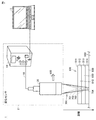•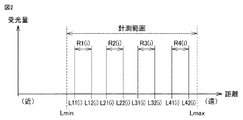••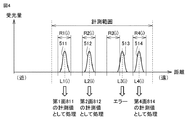•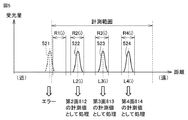••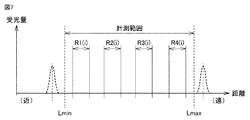•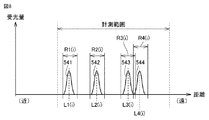••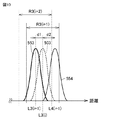•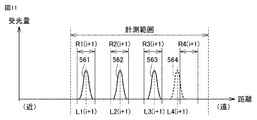•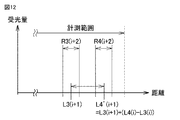•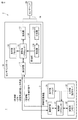•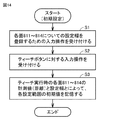•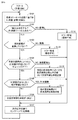•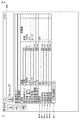•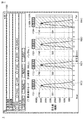•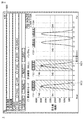•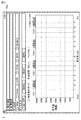•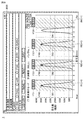•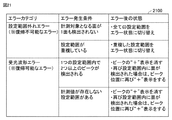•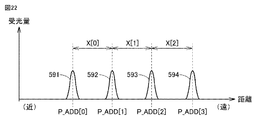•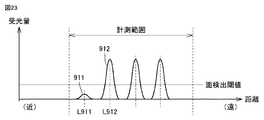•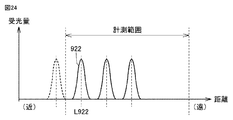## Classifications

• GPHYSICS
• G01MEASURING; TESTING
• G01BMEASURING LENGTH, THICKNESS OR SIMILAR LINEAR DIMENSIONS; MEASURING ANGLES; MEASURING AREAS; MEASURING IRREGULARITIES OF SURFACES OR CONTOURS
• G01B11/00Measuring arrangements characterised by the use of optical means
• G01B11/14Measuring arrangements characterised by the use of optical means for measuring distance or clearance between spaced objects or spaced apertures
• GPHYSICS
• G01MEASURING; TESTING
• G01BMEASURING LENGTH, THICKNESS OR SIMILAR LINEAR DIMENSIONS; MEASURING ANGLES; MEASURING AREAS; MEASURING IRREGULARITIES OF SURFACES OR CONTOURS
• G01B11/00Measuring arrangements characterised by the use of optical means
• G01B11/02Measuring arrangements characterised by the use of optical means for measuring length, width or thickness
• G01B11/06Measuring arrangements characterised by the use of optical means for measuring length, width or thickness for measuring thickness, e.g. of sheet material
• G01B11/0608Height gauges
• GPHYSICS
• G06COMPUTING; CALCULATING; COUNTING
• G06FELECTRIC DIGITAL DATA PROCESSING
• G06F17/00Digital computing or data processing equipment or methods, specially adapted for specific functions
• G06F17/10Complex mathematical operations
• G06F17/11Complex mathematical operations for solving equations, e.g. nonlinear equations, general mathematical optimization problems
• GPHYSICS
• G01MEASURING; TESTING
• G01BMEASURING LENGTH, THICKNESS OR SIMILAR LINEAR DIMENSIONS; MEASURING ANGLES; MEASURING AREAS; MEASURING IRREGULARITIES OF SURFACES OR CONTOURS
• G01B2210/00Aspects not specifically covered by any group under G01B, e.g. of wheel alignment, caliper-like sensors
• G01B2210/50Using chromatic effects to achieve wavelength-dependent depth resolution

## Abstract

A displacement measuring device capable of accurately measuring a distance to a surface to be measured is obtained. A displacement measuring device emits a light to a measurement target object, a light emitting unit that generates light, and receives the light reflected on the measurement target surface of the measurement object among the irradiated light. And a control unit that calculates a value indicating a distance to the surface to be measured based on light received by the sensor head. The control unit processes the calculated value as a value indicating the distance to the surface to be measured, on the condition that the calculated value is included in a preset numerical range, and the numerical value is calculated based on the calculated value. Reset the range. [Selected figure] Figure 4

## Description

The present invention relates to a displacement measuring device, a system including the displacement measuring device, and a displacement measuring method.

Conventionally, as shown in, for example, Patent Document 1, a displacement measurement apparatus using a white confocal method as a measurement method is known. The displacement measuring device continuously measures the distance (displacement) to the object to be measured while moving the non-contact type sensor head.

There is also a work in which quality control is performed by measuring the thickness or displacement of a part based on reflected light from a plurality of positions. As such a work, for example, a work including a material which is difficult to reflect light (optical parts having anti-reflection processing such as AR (Anti Reflective) coat, etc.), works (multi-lens group etc.) having a multilayer structure It can be mentioned.

Also, as shown in Patent Document 2, a displacement measuring device using a triangular distance measurement method as a measurement method is known.

JP 2012-208102 A JP, 2013-90045, A

In the work as described above, the reflected light from all the measurement positions may not be stable. For example, as shown as a waveform 911 in FIG. 23, when the light reception amount of light reflected by the first measurement target surface is small and not more than the surface detection threshold value, the displacement measuring device is shown as a waveform 912, 2 The light reflected by the first measurement target surface is treated as the light reflected by the first measurement target surface. Specifically, the displacement measurement device processes the distance to the first measurement target surface as the distance L912 instead of the distance L911.

In addition, when there is reflected light from many positions, the surface to be measured is out of the measurement range due to a slight positional deviation of the work. For example, as shown in FIG. 24, when the first surface to be measured is out of the measurement range, the displacement measuring device causes the light reflected by the second surface to be measured shown as waveform 922 to be the first light. It is treated as light reflected by the surface to be measured. Specifically, the displacement measuring device processes the distance to the first measurement target surface as the distance L922.

When the relative position between the sensor head and the work is successively shifted and the measurement is repeated, the positions of the waveforms 912, 922, etc. may fluctuate in the horizontal axis direction of FIGS.

Therefore, in the case of the work as described above, there may occur a case where the distance to each surface to be measured can not be accurately measured. Therefore, it is required to accurately measure the distance to the measurement target surface of various types of workpieces including such workpieces.

This indication is made in view of the above-mentioned problem, and the object is to provide a displacement measuring device, a system, and a displacement measuring method which can measure distance to a measurement object surface precisely.

According to an aspect of the present invention, the displacement measuring device emits a light to the light emitting unit that generates light and the measurement target, and reflects the light on the measurement target surface of the measurement target among the irradiated light. And a control unit configured to calculate a value indicating a distance to the surface to be measured based on the light received by the sensor head. The control unit processes the calculated value as a value indicating the distance to the surface to be measured on the condition that the calculated value is included in a predetermined numerical range, and the numerical value is calculated based on the calculated value. Reset the range.

According to the above configuration, it is possible to accurately measure the distance to each measurement target surface.

It is a figure showing the composition of the measurement system concerning this embodiment. It is a figure for demonstrating the setting range of each measurement object surface. It is a figure showing the i-th measurement value in a certain situation. It is a figure showing the i-th measurement value in the aspect different from FIG. It is a figure showing the i-th measurement value in the aspect different from FIG. 3 and FIG. It is a figure for demonstrating the change of a setting range. It is a figure showing the case where a sensor controller was not able to acquire measurement value. It is a figure showing the case where a setting range overlaps by change of a setting range. It is a figure showing the case where a plurality of measurement object sides were detected within one set range. It is a figure for demonstrating the method to set a setting range. It is a figure showing the case where a measured value is not in a setting range. It is a figure for demonstrating the method to set a setting range. It is a figure for demonstrating the functional structure of a measurement system. It is a flowchart showing the flow of processing at the time of initial setting. It is a flow figure showing the flow of measurement processing performed after initialization ends. It is a figure showing the screen displayed in the case of initial setting. It is a figure showing the screen of the measurement time in which measurement is normally performed. It is a figure showing the screen of the measurement time when a setting range overlaps. It is a figure showing the screen of the measurement time when it is judged in the sensor controller that the surface to be measured does not exist. It is a figure showing the screen in the measurement time when it is judged in the sensor controller that there is a setting range in which a measured value does not exist. It is the figure put together about the error. It is a figure for demonstrating the automatic setting of setting width. It is a figure showing the waveform detected by a displacement sensor. It is a figure showing the other waveform detected by the displacement sensor.

Hereinafter, embodiments of the present invention will be described with reference to the drawings. In the following description, the same components are denoted by the same reference numerals. Their names and functions are also the same. Therefore, detailed description thereof will not be repeated.

<A. System configuration>
FIG. 1 is a diagram showing the configuration of a measurement system according to the present embodiment.

Referring to FIG. 1, measurement system 1 includes displacement sensor 2 and information processor 3. The information processing device 3 is typically a personal computer.

The displacement sensor 2 is a sensor using wavelength dispersion. Specifically, the displacement sensor 2 is a displacement measurement device using a white confocal method as a measurement method. The displacement sensor 2 is also referred to as a fiber coaxial displacement sensor. The displacement sensor 2 includes a sensor controller 10, a light guide 19, and a sensor head 20. The sensor head 20 includes a confocal optical system. Specifically, the sensor head 20 has an objective lens and a chromatic aberration unit.

The sensor controller 10 generates light (typically white light) having a predetermined wavelength spread (wavelength width). The light propagates through the light guide 19 and reaches the sensor head 20.

In the sensor head 20, the propagated light is focused by the objective lens and irradiated to the measurement object 800. In the irradiation light 700, axial chromatic aberration occurs by passing through the chromatic aberration unit, so the focal position of the irradiation light irradiated from the objective lens differs depending on the wavelength. Only the light of the wavelength focused on the measurement object 800 will be re-incident on the light guide section 19.

The measurement object 800 has a plurality of layers 810, 820, 830 capable of transmitting white light. Each of these layers 810, 820, 830 is formed on, for example, a substrate 840 (base material) which does not transmit white light.

Light 701 of a wavelength focused on the first surface 811 of the measurement object 800, light 702 of a wavelength focused on the second surface 812, and light 703 of a wavelength focused on the third surface 813; The light 704 having a wavelength focused on the fourth surface 814 is received by the sensor head 20 as reflected light.

Each of the surfaces 811 to 814 is a surface to be measured. Specifically, the first surface 811 is an upper surface of the first layer 810 and is a surface exposed to the outside. The second surface 812 is an upper surface of the second layer 820 and in contact with the upper layer 810. The third surface 813 is an upper surface of the third layer 830 and in contact with the upper layer 820. The fourth surface 814 is a surface of the substrate 840, which is in contact with the upper layer 830.

Reflected light received by the sensor head 20 is incident on the sensor controller 10 via the light guide 19. The sensor controller 10 calculates the distance from the sensor head 20 to the measurement target 800 based on the reflected light. Specifically, the sensor controller 10 calculates each distance (displacement) from the sensor head 20 to each layer 810, 820, 830 of the measurement object 800 and the distance (displacement) to the substrate 840 based on the reflected light. Do.

In the displacement sensor 2, the measurement of the distance and the movement of the sensor head 20 in the direction of, for example, the arrow 600 are repeated. Thereby, the measured value in the several location along a surface is obtained about each of the surfaces 811-814.

The measurement object 800 may move on the production line without moving the sensor head 20. The present embodiment is applicable to a system in which measurement is performed while the relative position between the sensor head 20 and the measurement object 800 changes.

The information processing device 3 is connected to the sensor controller 10. The information processing device 3 can change various settings of the sensor controller 10. Further, in the information processing device 3, information such as the distance calculated by the sensor controller 10 can be displayed. Various functions of the information processing device 3 will be described later. Such setting and display can be performed by the sensor controller 10 alone.

The hardware configurations of the sensor controller 10, the sensor head 20, and the light guide 19 are the same as conventional ones, and therefore the description thereof will not be repeated here.

<B. Outline of processing>
In the measurement system 1, the setting range of each surface 811 to 814 is designated, and the distance obtained within each setting range is set as the distance by each of the surfaces 811 to 814. Note that the setting range can also be said to be a region that defines the position of the measurement target surface (that is, the distance at which the light reception amount reaches a peak). Hereinafter, a specific example of such a configuration will be described.

FIG. 2 is a diagram for explaining the setting range of the surfaces 811 to 814. As shown in FIG.
Referring to FIG. 2, a range R1 (i) is designated as the setting range of the first surface 811. Similarly, a range R2 (i) is designated as the setting range of the second surface 812. Further, a range R3 (i) is designated as a setting range of the third surface 813, and a range R4 (i) is designated as a setting range of the fourth surface 814. Details of this range setting method will be described later.

The range R1 (i) is a range from the distance L11 (i) to the distance L12 (i). Likewise, the ranges R2 (i), R3 (i) and R4 (i) are respectively from the distance L21 (i) to the distance L22 (i) and from the distance L31 (i) to the distance L32 (i) Of the distance L41 (i) to the distance L42 (i). The range (measurement range) that can be measured by the displacement sensor 2 is a range from the distance Lmin to Lmax.

In the above, i is one or more arbitrary natural numbers. The initial values (R1 (1), R2 (1), R3 (1), R4 (1)) of each range R1 (i) to R4 (i) are set by the user using the information processing device 3. . In addition, the displacement sensor 2 sequentially changes the ranges R1 (i), R2 (i), R3 (i) and R4 (i) for each measurement cycle. That is, focusing on the range R1 (i), R1 (i + 1) is set (re-set) after R1 (i), and R1 (i + 2) is set (re-set) after that. Such change (re-setting) of the setting range is performed by the control unit 11 (see FIG. 13) of the sensor controller 10 configuring the displacement sensor 2.

Hereinafter, the ranges R1 (i), R2 (i), R3 (i), and R4 (i) will be described as setting ranges used for the i-th measurement. In addition, since measurement and movement of the sensor head 20 are performed repeatedly, the i-th measurement point and the i + 1-th measurement point are different.

FIG. 3 is a diagram showing the i-th measurement value in a certain phase.
Referring to FIG. 3, in the sensor controller 10, waveforms 501, 502, 503, and 504 of four wavelengths are detected as a waveform indicating the amount of received reflected light. The distances at which the waveforms 501, 502, 503, and 504 peak are the distances L1 (i), L2 (i), L3 (i), and L4 (i), respectively.

The distance L1 (i) is included in the range R1 (i). Therefore, the sensor controller 10 treats the distance L1 (i) as the distance to the first surface 811 of the layer 810. Specifically, the distance L1 (i) is determined as a measurement value.

Further, the distance L2 (i) is included in the range R2 (i). Thus, the sensor controller 10 treats the distance L2 (i) as the distance to the second surface 812 of the layer 820. Similarly, the sensor controller 10 treats the distance L3 (i) as the distance to the third surface 813 and treats the distance L4 (i) as the distance to the fourth surface 814.

FIG. 4 is a diagram showing an i-th measurement value in a phase different from that in FIG. 3.
Referring to FIG. 4, in the sensor controller 10, waveforms 511, 512, 513, and 514 of four wavelengths are detected as a waveform indicating the amount of received reflected light. The peaks of the waveforms 511, 512, 513, and 514 are described as distances L1 (i), L2 (i), L3 (i), and L4 (i), respectively, as in FIG. 3 for convenience of explanation.

The distance L1 (i) at which the amount of received light peaks in the waveform 511 is included in the range R1 (i). Therefore, the sensor controller 10 processes the distance L1 (i) as the distance to the first surface 811. Similarly, the sensor controller 10 treats the distance L2 (i) as the distance to the second surface 812 and treats the distance L4 (i) as the distance to the fourth surface 814.

However, the distance L3 (i) at which the amount of received light peaks in the waveform 513 is not included in the range R3 (i). For this reason, the sensor controller 10 does not process the distance L3 (i) as the distance to the third surface 813. The sensor controller 10 processes (error processing) the distance to the third surface 813 as unmeasurable.

As described above, the sensor controller 10 is configured to set the distance included in the predetermined numerical range (for example, the distance L1 (i) included in the range R1 (i)) to the plurality of layers among the four calculated distances. It is treated as a distance to a surface to be measured of a predetermined layer of 810, 820, 830 and substrate 840 or a surface to be measured of substrate 840 (for example, a distance to first surface 811 of layer 810).

According to such a configuration, since the distance not included in the numerical range is not processed as the distance to the measurement target surface, the distance to each of the surfaces 811 to 814 can be accurately measured.

FIG. 5 is a diagram showing an i-th measurement value in a phase different from FIGS. 3 and 4.
Referring to FIG. 5, in the sensor controller 10, waveforms 521, 522, 523 and 524 of four wavelengths are detected as waveforms showing the amount of received reflected light. The distances at which the waveforms 522, 523 and 524 become peaks are described as L2 (i), L3 (i) and L4 (i) for convenience of explanation, as in FIG. 3 and FIG.

The distance L2 (i) at which the amount of received light peaks in the waveform 522 is included in the range R2 (i). Therefore, the sensor controller 10 processes the distance L 2 (i) as the distance to the second surface 812. Similarly, the sensor controller 10 treats the distance L3 (i) as the distance to the third surface 813 and treats the distance L4 (i) as the distance to the fourth surface 814.

However, since the first surface 811 is at a position outside the measurement range of the sensor head 20 as the waveform 521 shows, the sensor controller 10 can not detect the peak of the light reception amount included in the range R1 (i). Therefore, the sensor controller 10 processes (error processing) the distance to the first surface 811 as unmeasurable.

As described above, the sensor controller 10 can set the distance within the predetermined numerical range among the calculated four distances to a predetermined number of the plurality of layers 810, 820, 830 and the substrate 840. It is processed as the distance to the surface to be measured or the surface to be measured of the substrate 840.

According to such a configuration, the distance not included in the numerical range is not processed as the distance to the surface to be measured. In particular, in the case of FIG. 5, the sensor controller 10 can prevent the distance to the first surface 811 which is the first measurement target surface from being processed as the distance L2 (i). Therefore, according to the sensor controller 10, the distances to the surfaces 811 to 814 can be measured with high accuracy.

FIG. 6 is a diagram for explaining the change of the setting range.
With reference to FIG. 6, the sensor controller 10 changes the setting range of the first surface 811 from the range R1 (i) to the range R (i + 1) based on the distance L1 (i) included in the range R1 (i). change. Typically, the sensor controller 10 sets (resets) the range R1 (i + 1) such that the distance L1 (i) becomes the middle value of the range R1 (i + 1).

Similarly, the sensor controller 10 changes the setting range regarding the second surface 812 from the range R2 (i) to the range R2 (i + 1) based on the distance L2 (i) included in the range R2 (i). Further, the sensor controller 10 changes the setting range for the third surface 813 from the range R3 (i) to the range R3 (i + 1), and the setting range for the fourth surface 814 ranges from the range R4 (i) to the range R4 (i.e. Change to i + 1).

As described above, the displacement sensor causes the setting range of each of the surfaces 811 to 814 to follow the distances L1 (i) to L4 (i) which are measurement values. According to this, the number of times the error processing is performed is reduced as compared with a configuration in which such follow-up processing (setting range resetting processing) is not performed. Therefore, according to such a configuration, it is possible to accurately measure the distances to the surfaces 811 to 814, and to obtain many data on the distances to the surfaces 811 to 814.

In the above configuration, although the configuration for continuing measurement even when an error occurs is described as an example, the measurement system 1 may be configured to stop measurement when an error occurs. Good.

<C. Processing Details>
(C1. Initial setting)
As described above, the ranges R1 (1), R2 (1), R3 (1), R4 (1), which are initial values, are set by the user using the information processing apparatus 3. Such an initial value is set in the teaching mode. Such setting can also be performed by the sensor controller 10 alone.

The sensor controller 10 typically stores in advance a default value of a numerical range. This default value is changed based on the teaching process, and the changed value is used as the initial value.

The sensor controller 10 measures the distance to each surface 811, 812, 813, 814 measured at the time of teaching (the distance of the peak of the received light amount) and the width of the setting range input by the user (upper limit value and lower limit value of setting range) The ranges R1 (1), R2 (1), R3 (1), R4 (1) are set based on the difference). Specifically, the sensor controller 10 determines the range R1 (1) such that the distance to the first surface 811 becomes the middle value of the input width. The sensor controller 10 sets the range R2 (1) such that the distance to the second surface 812 is the middle value of the input width. The same method is set for the ranges R3 (1) and R4 (1).

In the measurement system 1, the width of the setting range can be set for each of the ranges R1 (1), R2 (1), R3 (1), R4 (1).

(C2. Processing after start of measurement)
At the time of measurement, various aspects can occur in addition to the aspects shown in FIG. 3, FIG. 4 and FIG. Hereinafter, processing in the case where a phase other than the above-described phase occurs will be described.

(1) When a Measurement Value Can not be Obtained FIG. 7 is a diagram showing a case where the sensor controller 10 can not obtain a measurement value.

Referring to FIG. 7, when there is no peak of the light reception amount in the measurement range (within the range of Lmin to Lmax), the sensor controller 10 determines that there is no surface to be measured, and it deviates from all setting ranges. Treat as an error. In this case, it is assumed that the sensor controller 10 does not have data representing the distances to the surfaces 811 to 814 for this measurement cycle.

(2) When the Setting Ranges Overlap FIG. 8 is a diagram showing the case where the setting ranges overlap due to the change of the setting ranges.

Referring to FIG. 8, it is assumed that waveforms 541, 542, 543, and 544 of four wavelengths are detected in sensor controller 10 after ranges R1 (i) to R4 (i) are set. The distances at which the waveforms 541, 542, 543, and 544 peak are assumed to be distances L1 (i), L2 (i), L3 (i), and L4 (i), respectively.

The distance L1 (i) at which the amount of received light peaks in the waveform 541 is included in the range R1 (i). Therefore, the sensor controller 10 treats the distance L1 (i) as the distance to the first surface 811 of the layer 810. Similarly, the sensor controller 10 treats the distance L 2 (i) as the distance to the second surface 812.

However, the range R3 (i) and the range R4 (i) which have already been set partially overlap each other. In such a case, the sensor controller 10 treats the measurement values included in the overlapping range as an error. In this case, the sensor controller 10 does not have the measurement value of the third surface 813 (distance L3 (i)) and the measurement value of the fourth surface 814 (distance L4 (i)) for this measurement cycle. Do.

(3) When a plurality of measurement target surfaces are detected in one set range FIG. 9 is a view showing a case where a plurality of measurement target surfaces are detected in one set range.

Referring to FIG. 9, it is assumed that, after ranges R1 (i + 1) to R4 (i + 1) are set, the sensor controller 10 detects waveforms 551, 552, 553, and 554 of four wavelengths. The distances at which the waveforms 551, 552, 553, and 554 become peaks are assumed to be distances L1 (i + 1), L2 (i + 1), L3 (i + 1), and L4 (i + 1), respectively.

The distance L1 (i + 1) at which the light reception amount peaks in the waveform 551 is included in the range R1 (i + 1). Therefore, the sensor controller 10 treats the distance L1 (i + 1) as the distance to the first surface 811 of the layer 810. Similarly, the sensor controller 10 processes the distance L 2 (i + 1) as the distance to the second surface 812.

However, the range R3 (i + 1) includes two peaks of the light reception amount. That is, the sensor controller 10 detects two measurement target surfaces in the range R3 (i + 1).

In such a case, the sensor controller 10 sets a range R3 (i + 2) to be set next to the range R3 (i + 1) as follows.

FIG. 10 is a diagram for describing a method of setting the range R3 (i + 2).
Referring to FIG. 10, the sensor controller 10 selects one of the distance L3 (i + 1) and the distance L4 (i + 1) that is closer to the previous measurement value (the distance L3 (i)). If the distance between the distance L3 (i) and the distance L3 (i + 1) is d1 and the distance between the distance L3 (i) and the distance L4 (i + 1) is d2 (> d1), then the sensor The controller 10 selects the distance L3 (i + 1). In this case, the sensor controller 10 sets the range R3 (i + 2) such that the distance L3 (i + 1) becomes the middle value of the range R3 (i + 2) to be set next.

Thus, when the distance L3 (i + 1) and the distance L4 (i + 1) are included in the range R3 (i + 1), the sensor controller 10 determines the distance L3 (i) of the distance L3 (i + 1) and the distance L4 (i + 1). So that the distance closer to is the middle value of the changed range R3 (i + 2).

According to such a configuration, the following range can be set appropriately.
(4) When the Measured Value is not in the Set Range FIG. 11 is a diagram showing the case where the measured value is not in the set range.

Referring to FIG. 11, it is assumed that four wavelength waveforms 561, 562, 563, and 564 are detected in the sensor controller 10 after the ranges R1 (i + 1) to R4 (i + 1) are set. The distances at which the waveforms 561, 562, 563, 564 peak are assumed to be distances L 1 (i + 1), L 2 (i + 1), L 3 (i + 1), and L 4 (i + 1), respectively.

The distance L1 (i + 1) at which the light reception amount peaks in the waveform 561 is included in the range R1 (i + 1). Therefore, the sensor controller 10 treats the distance L1 (i + 1) as the distance to the first surface 811 of the layer 810. Similarly, the sensor controller 10 processes the distance L2 (i + 1) as the distance to the second surface 812 and processes the distance L3 (i + 1) as the distance to the third surface 813.

However, the distance L4 (i + 1) at which the amount of received light peaks for the waveform 564 is not included in R4 (i + 1). For this reason, the sensor controller 10 does not process the distance L4 (i + 1) as the distance to the fourth surface 814. The sensor controller 10 processes (error processing) the distance to the fourth surface 814 as unmeasurable.

In such a case, the sensor controller 10 sets a range R4 (i + 2) to be set next to the range R4 (i + 1) as follows.

FIG. 12 is a diagram for describing a method of setting the range R4 (i + 2).
Referring to FIG. 12, sensor controller 10 uses distance L3 (i), distance L3 (i + 1), and distance L4 (i) to determine range L4 (i + 2). Calculate i + 1). The sensor controller 10 does not use the distance L4 (i + 1) to determine the range R4 (i + 2).

Specifically, the sensor controller 10 calculates the distance L4 '(i + 1) by the following equation (1).

L4 '(i + 1) = L3 (i + 1) + (L4 (i) -L3 (i)) (1)
Thus, the sensor controller 10 calculates the distance L4 '(i + 1) using the relative positional relationship between the two measurement values in the previous measurement. The sensor controller 10 sets the range R4 (i + 2) such that the distance L4 '(i + 1) becomes the middle value of the range R4 (i + 2) to be set next.

As described above, in the sensor controller 10, at least one of the plurality of previous measurement values (L1 (i + 1), L2 (i + 1), L3 (i + 1), L4 (i + 1)) is included in the range R4 (i + 1). If not, the setting range for the fourth surface 814 is set to the range R4 (L4 (i), the distance L3 (i), and the distance L3 (i + 1) included in the range R3 (i + 1). Change from i + 1) to the range R4 (i + 2).

According to such a configuration, the range R4 (i + 2) can be set with high accuracy even when the range R4 (i + 2) is set using the distance L4 (i + 1) shown in FIG.

Although L3 (i + 1) and L3 (i) are used to set the range R4 (i + 2) in the above description, L2 (i + 1) and L2 (i) are used instead. May be Alternatively, the sensor controller 10 may use L1 (i + 1) and L1 (i).

(C3. Functional configuration)
FIG. 13 is a diagram for explaining the functional configuration of the measurement system 1.

Referring to FIG. 13, measurement system 1 includes displacement sensor 2 and information processor 3 as described above. The displacement sensor 2 includes a sensor controller 10, a light guide 19, and a sensor head 20.

The sensor controller 10 includes a control unit 11, a light emitting unit 12, a light receiving unit 13, a display unit 14, and a communication IF (Inter Face) unit 15. The light receiving unit 13 includes a spectroscope 131 and a detector 132.

The irradiation light having a predetermined wavelength spread generated by the light projecting unit 12 propagates through the light guiding unit 19 and reaches the sensor head 20. The reflected light re-incident on the sensor head 20 propagates through the light guide 19 and is incident on the light receiver 13. In the light receiving unit 13, the reflected light incident by the spectroscope 131 is separated into each wavelength component, and the intensity of each wavelength component is detected by the detector 132.

The control unit 11 calculates the distance (displacement) from the sensor head 20 to the surfaces 811 to 814 of the measurement object 800 based on the detection result of the detector 132. Further, the control unit 11 changes (re-sets) the setting range of each of the surfaces 811 to 814.

The display unit 14 displays the distance calculated by the control unit 11 numerically.
Among the plurality of light receiving elements constituting the detector 132 of the light receiving unit 13, the light receiving element that receives the reflected light changes in accordance with the shape of the surface of the measurement object 800 with respect to the sensor head 20. Therefore, the distance change (displacement) with respect to each surface 811 to 814 of the measurement object 800 can be measured from the detection results (pixel information) by the plurality of light receiving elements of the detector 132. Thereby, the shape of each measurement target surface of the measurement object 800 can be measured by the displacement sensor 2.

The communication IF unit 15 is used for communication with the information processing device 3.
The information processing device 3 includes a control unit 31, a storage unit 32, an input unit 33, a display unit 34, and a communication IF unit 35.

The control unit 31 controls the operation of the information processing device 3. The control unit 31 executes a predetermined application program on the operating system stored in the storage unit 32. An example of the screen (user interface) displayed on the display unit 34 by the execution of the application program will be described later.

The control unit 31 receives a user input (input operation) via the input unit 33. Further, the control unit 31 outputs the screen to the display unit 34. The control unit 31 communicates with the sensor controller 10 via the communication IF unit 35.

The information processing device 3 transmits, to the sensor controller 10, information on the setting width of the setting range and a teach execution instruction used in the initial setting. The setting width is a value input using the input unit 33. The set width is, for example, a value obtained by subtracting L11 (i) from L12 (i) in the case of the range R1 (i) in FIG.

Further, the teaching execution instruction is transmitted from the information processing device 3 to the sensor controller 10 by the user selecting a predetermined object (hereinafter, also referred to as a “teach button”) displayed on the display unit 34.

The sensor controller 10 transmits measurement values to the information processing device 3 each time measurement is performed, as an example. The measurement value processed as an error is not transmitted to the information processing device 3. Further, in the case of an error, the sensor controller 10 notifies the information processing device 3 to that effect. Furthermore, the sensor controller 10 sets the value (distance) of the middle value of the setting range each time the setting range (range R1 (i) to R4 (i)) for each surface 811 to 814 is changed (reset) Is notified to the information processing apparatus 3.

The information processing device 3 updates and displays the measurement value (waveform) and the like on the display unit 34 based on the received various data. By the user visually recognizing the screen of the display unit 34, it is possible to confirm temporal changes and the like of the waveform.

(C4. Control structure)
FIG. 14 is a flow diagram showing the flow of processing at the time of initial setting.

Referring to FIG. 14, in step S <b> 1, the information processing device 3 receives an input operation (user input) for registering the setting widths of the surfaces 811 to 814. When the set width is input, in step S2, the information processing device 3 receives an input operation on the teach button. Thereby, the information of the setting width is sent from the information processing device 3 to the sensor controller 10.

In step S3, the sensor controller 10 performs each setting based on the measurement values (distances) of the surfaces 811 to 814 during teaching execution and the information of the setting widths of the surfaces 811 to 814 received from the information processing device 3. The initial value of the range (R1 (1), R2 (i), R3 (i), R4 (i)) is stored.

FIG. 15 is a flowchart showing the flow of measurement processing executed after the initial setting is completed.

Referring to FIG. 15, in step S101, the sensor controller 10 calculates measurement values (distances) based on the positions of the surfaces 811 to 814. In step S102, the sensor controller 10 determines whether or not one or more measurement target surfaces exist based on the calculation result. That is, the sensor controller 10 determines whether there are one or more measurement values.

If it is determined that one or more planes exist (YES in step S102), the sensor controller 10 determines in step S103 whether or not the setting ranges overlap.

If the sensor controller 10 determines that there is no one side (NO in step S102), the sensor controller 10 executes an error process with no face in step S108. Specifically, as described with reference to FIG. 7, the sensor controller 10 determines that the surface to be measured does not exist, and processes the error as an error out of the entire setting range. Specifically, the sensor controller 10 assumes that there is no data representing the distance to each of the surfaces 811 to 814 with respect to this measurement time.

When the sensor controller 10 determines that the setting ranges do not overlap (YES in step S103), in step S104, the sensor controller 10 determines whether two or more measurement values exist in each setting range. That is, the sensor controller 10 determines whether there are zero or only one measured value included in one set range.

If the sensor controller 10 determines that the setting ranges overlap (NO in step S103), the sensor controller 10 performs error processing as setting range overlapping in step S109. Specifically, as described with reference to FIG. 8, the sensor controller 10 measures the measurement values of the respective surfaces included in the overlapping setting range (in the case of FIG. It is assumed that the distances L3 (i) and L4 (i) do not exist.

If the sensor controller 10 determines that two or more measurement values do not exist in each setting range (YES in step S104), whether or not there is a setting range in which no measurement values exist in step S105. To judge.

When the sensor controller 10 determines that two or more measurement values do not exist in each setting range (NO in step S104), the previous measurement is performed in step S110 as described based on FIG. 9 and FIG. The setting range for the next measurement cycle is set with the value closer to the position as the middle value of the setting range.

When it is determined that the measurement value exists in each setting range (NO in step S105), the sensor controller 10 resets each setting range in step S106 as described based on FIG. 6, for example.

When the sensor controller 10 determines that there is a setting range in which no measurement value exists (YES in step S105), in step S111, as described based on FIGS. 11 and 12, the setting in which no measurement value exists About a range, a setting range is changed using the measured value of the setting range in which a measured value exists in a setting range. Moreover, the setting range is changed using the said measured value also about the setting range in which the measured value exists in a setting range.

In step S107, the sensor controller 10 moves the sensor head 20 to the next measurement position as indicated by the arrow 600 in FIG.

<D. User interface>
Next, a screen example displayed on the display unit 34 (see FIG. 13) of the information processing device 3 will be described. The display of the screen is performed by the control unit 31.

(D1. Setting screen)
FIG. 16 is a diagram showing a screen displayed at the time of initial setting.

Referring to FIG. 16, at least a check box 1610, setting items 1611, 1612, 1613, and 1614 and an object 1621 are displayed on the screen 1600.

The check box 1610 is used to determine whether to set the surface to be measured. The setting item 1611 is used to input the setting width of the first surface 811. The setting item 1612 is used to input the setting width of the second surface 812. The setting item 1613 is used to input the setting width of the third surface 813. The setting item 1614 is used to input the setting width of the fourth surface 814.

The object 1621 is an object for the user to instruct execution of teaching.

(D2. Normal processing)
FIG. 17 is a diagram showing a screen of measurement times in which measurement is normally performed. FIG. 17 is also a diagram corresponding to the state of FIG.

Referring to FIG. 17, control unit 31 displays a screen 1700 including at least markers 1721, 1722, 1723 and 1724, waveforms 501 to 504, and four cross markers 1740.

The control unit 31 displays the inside of the set range in a manner different from the other ranges. For example, the control unit 31 sets the background color in the setting range to be different from the background colors in the other ranges. Specifically, the control unit 31 sets the setting range (ranges R1 (i), R2 (i), R3 (i), R4 (i)) to be green, thereby setting white as a color in another range. Make it different.

The marks 1721, 1722, 1723 and 1724 are for visually identifying each of the four setting areas. Control unit 31 moves the positions of markers 1721, 1722, 1723 and 1724 to the left and right in accordance with the change of the corresponding setting range. Typically, the control unit 31 causes the positions of the marks 1721, 1722, 1723 and 1724 to be at the middle position of the corresponding setting range.

The cross mark 1740 is displayed at the position of the peak of the waveforms 501 to 504.
(D3. When overlapping setting range: NO in step S103)
FIG. 18 is a diagram showing a screen of measurement times when the setting range is overlapped. FIG. 18 is also a diagram corresponding to the state of FIG.

Referring to FIG. 18, control unit 31 displays screen 1800 including at least markers 1721 to 1724, waveforms 541 to 544, and two cross markers 1740.

The difference between the screen 1700 shown in FIG. 17 and the screen 1700 will be described. In order to visually notify the user of the overlapping on the screen 1800, the setting range (R3 (i)) of the third surface 813 and For the setting range (R4 (i)) of the fourth surface 814, the color arrangement such as green is not performed.

In addition, the control unit 31 displays the markers 1723 and 1724 for the measurement target surface in which the setting range is overlapped in a mode different from the markers 1721 and 1722 for the non-overlapping measurement target surface. For example, the control unit 31 may change the entire color of the markers 1723 and 1724 or the color of part of the markers (for example, a frame) from the color of the markers 1721 and 1722 (hereinafter also referred to as “default color”). I assume.

Further, the control unit 31 processes the measurement value (distance) based on the waveforms 543 and 544 as an error (does not determine the measurement value), and therefore, in order to visually notify the user, the cross mark 1740 has the waveform 543 and 544. Do not display on the peak.

(D3. No surface to be measured: NO in step S102)
FIG. 19 is a diagram showing a screen of measurement times when it is determined in the sensor controller 10 that no surface to be measured exists. FIG. 19 is also a diagram corresponding to the state of FIG. 7.

Referring to FIG. 19, control unit 31 displays a screen 1900 including at least markers 1721 to 1724. However, the control unit 31 does not display all the setting ranges in green or the like, in order to notify the user that each of all the setting ranges does not include the waveform peak.

In addition, the control unit 31 sets each of the markers 1721 to 1724 to a color different from the default color as described in FIG. 18. Furthermore, as an example, the position of each of the markers 1721 to 1724 is set as the default position.

(D4. When there is a setting range in which no measured value exists: In the case of YES at step S105)
FIG. 20 is a diagram showing a screen in the measurement cycle when it is determined in the sensor controller 10 that there is a setting range in which no measurement value exists. Note that FIG. 20 is also a diagram corresponding to the state of FIG.

Referring to FIG. 20, control unit 31 displays screen 2000 including at least markers 1721 to 1724, waveforms 561 to 564 and four cross markers 1740.

Since the control unit 31 determines that the setting ranges do not overlap and that the measurement target surface exists, all the setting ranges are different from the other ranges, as in FIG. 17. indicate. However, since the peak of the waveform 564 is out of the range R4 (i + 1), the control unit 31 does not display the cross mark 1740 at the peak of the waveform 564 from the viewpoint of notifying the user that the waveform is out of the range.

(D5. Summary of errors)
FIG. 21 is a diagram summarizing the errors described above.

Referring to FIG. 21, there are an out-of-setting range error and a light reception waveform error as error categories.

The first condition of occurrence of the out-of-set range error is that even one surface to be measured as shown in FIG. 7 is not detected (case of NO in step S102 of FIG. 15). Further, the second condition of occurrence of the out-of-setting range error is that the setting range is overlapped as shown in FIG. 8 (in the case of NO in step S103 of FIG. 15).

One of the generation conditions of the light reception waveform error is that two or more peaks are detected in one set range as shown in FIG. 9 (NO in step S104 of FIG. 15). Further, the second condition of the occurrence of the light reception waveform error is that there is a setting range in which no measured value exists (NO in step S105 of FIG. 15).

The display content of the screen on the information processing device 3 corresponding to each error occurrence condition is described in the column of the state after the error. These descriptions correspond to FIG. 17, FIG. 18, FIG. 19, and FIG. 20 in order from the top.

The sensor controller 10 treats an out-of-setting error as an error that can not be recovered. On the other hand, the sensor controller 10 processes the light reception waveform error as an error that can be recovered.

There is no problem in processing the measured value as the distance to the surface to be measured if the measurement value falls within the set range (distance of the peak of the waveform) when the measurement is performed again at the same position. , It is classified as an error that can be recovered.

The out-of-set range error is classified as an unrecoverable error because it is not appropriate to process the measured value as the distance to the surface to be measured even if the measured value is included in the set range. In the case of an error outside the set range, the sensor controller 10 moves the position of the sensor head 20 to the next measurement position without performing measurement again.

E. Modified example>
(1) Displacement Measurement Device In the above, a displacement measurement device using a white confocal method has been described as the displacement sensor 2. That is, in the above, a measurement object having, as the displacement sensor 2, (i) a light projecting unit (light source) generating light having a predetermined wavelength spread, and (ii) a plurality of layers capable of transmitting light. On the other hand, an irradiation unit (part of a sensor head) which irradiates light so as to focus light at different positions on the optical axis for each wavelength, and (iii) focuses on each measurement target surface of a plurality of layers A light receiving unit that receives light of each wavelength that is connected and reflected and that detects each wavelength from the received light, and (iv) a measurement target for each wavelength (each measurement target surface) based on each detected wavelength And the control part which calculates the distance between and the example of the displacement measurement apparatus of a structure provided and demonstrated.

However, the displacement measuring device is not limited to such a configuration. For example, the processing described above in the control unit 11 can be applied to a displacement sensor (displacement measuring device) using a triangular distance measurement method.

In a displacement sensor using such a triangular distance measurement method, a part of light returning irregularly from the object to be measured is collected by a lens in the displacement sensor. Thereby, an image is formed on a position sensor (imaging element) such as a complementary metal oxide semiconductor (CMOS) in the displacement sensor. When the distance to the object to be measured changes, the light receiving position on the position sensor also changes. Therefore, the control unit 11 can obtain the distance to the measurement object by specifying the light receiving position on the position sensor.

As described above, the displacement measurement device emits light to the measurement object having at least (a) a light emitting unit that generates light and (b) a plurality of layers capable of transmitting light, and irradiation Calculate the distance between each measurement target surface based on the sensor head that receives the light reflected on each measurement target surface of each of the plurality of layers among the received light and the light received by the sensor head (c) And (d) the control unit includes, among the calculated distances, a first distance included in a predetermined numerical range (for example, a first setting range), among the plurality of layers. It may be configured to be processed as the distance to the measurement target surface of the predetermined layer (for example, the first layer).

Furthermore, in such configurations (c) and (d), in the case of the triangulation method, the control unit, instead of the distance, receives the light receiving position on the position sensor (specifically, Value) is calculated, and among the plurality of calculated pixel values, the first pixel value included in the predetermined numerical range is used as the pixel value up to the measurement target surface of the predetermined layer of the plurality of layers. It may be a configuration to be processed.

In the above configurations (c) and (d), in the case of the white confocal method, the control unit calculates the wavelength of light instead of the distance, and a predetermined one of the calculated wavelengths is used. The first wavelength included in the numerical range may be processed as the wavelength up to the measurement target surface of a predetermined layer among the plurality of layers.

As mentioned above, the control part should just be the composition which computes the value (distance, pixel value, wavelength) which shows distance. That is, of the values indicating the plurality of distances calculated, the control unit indicates the first value included in the predetermined numerical range as a value indicating the distance to the measurement target surface of the predetermined layer among the plurality of layers. As long as it is processed as

(2) Measurement Object In the above, as the measurement object, the measurement object 800 including the plurality of layers 810, 820, 830, each of which can transmit light (typically white light), is exemplified. However, the measurement object is not limited to such a configuration.

The measurement object may be configured to have only one layer (for example, layer 830), for example. That is, the layer transmitting light may be a single layer.

In addition, the measurement object may not include such a layer (a layer that transmits light). Only one measurement target surface may be measured.

(3) Setting Range In the above, in the teaching mode, the information processing device 3 or the sensor controller 10 sets the initial values R1 (1), R2 (1), R3 (1) in the respective ranges R1 (i) to R4 (i). And R4 (1) have been described as an example, but the present invention is not limited thereto. The user may be configured to determine (set) the initial values R1 (1), R2 (1), R3 (1) and R4 (1).

Further, in the above description, the ranges R1 (i) to R4 (i) are respectively provided for the four measurement target surfaces (the first surface 811, the second surface 812, the third surface 813 and the fourth surface 814). However, the present invention is not limited to this. The determination process using the range may be performed on three or less measurement target surfaces based on the user operation. That is, the information processing apparatus 3 or the sensor controller 10 may be configured such that the user can designate at least one setting range of the surfaces 811 to 814.

In this manner, the user input for designating the numerical range corresponding to one or more measurement target surfaces of the plurality of measurement target surfaces in association with the measurement target surface is received, and included in the numerical range The information processing device 3 or the sensor controller 10 may be configured to process a certain value as a value indicating the distance to the measurement target surface corresponding to the numerical value range.

For example, among four initial values R1 (1), R2 (1), R3 (1), R4 (1), user input for specifying a numerical range of R1 (1) and R2 (1) is accepted, The value included in R1 (1) is processed as a value indicating the distance to the first surface 811 corresponding to the numerical value range, and the value included in R2 (1) is corresponding to the numerical value range The information processing device 3 or the sensor controller 10 may be configured to process as a value indicating the distance to the second surface 812. In this case, the distance to the third surface 813 and the fourth surface 814 does not have a range designation, and thus is the value calculated by the information processing device 3 or the sensor controller 10 itself.

(Automatic setting of setting width)
In the above, the configuration for receiving the user input of the width of the setting range (setting width) has been described as an example, but the sensor controller 10 or the information processing device 3 may automatically calculate the optimum setting width. Hereinafter, such a configuration will be described based on FIG. The setting width is not dynamically changed, and the same value is used during measurement.

FIG. 22 is a diagram for describing the automatic setting of the setting width. Referring to FIG. 22, it is assumed that sensor controller 10 detects waveforms 591, 592, 593, 594 of four wavelengths.

In the following, the variable N is taken as the number of faces. When N is 0, no face detection is performed. Also, in this example, the maximum value of N is four. The variable X [n] is the relative distance (in units of pixels) of each surface. The unit may be mm. When calculating with pixel values, it is finally necessary to convert to mm values. The variable TW [n] is a set width (edge track width). n is a natural number greater than or equal to 2 and less than or equal to N-1.

Here, it is assumed that the peaks of the waveforms 591, 592, 593, 594 of the four wavelengths are P_ADD , P_ADD , P_ADD , and P_ADD , respectively. The unit is a pixel.

In this case, the sensor controller 10 sets the setting width of the first surface 811, the setting width of the second surface 812, the setting width of the third surface 813, and the setting width of the fourth surface 814 as follows. Do.

(A) Setting width of the first surface 811 = X  / 4
(B) Setting width of the second surface 812 = X  / 4 (when X  / 4> X  / 4)
X  / 4 (other than the above)
(C) Setting width of the third surface 813 = X  / 4 (when X  / 4> X  / 4)
X  / 4 (other than above)
(D) Setting width of fourth surface 814 = X  / 4
Thus, the sensor controller 10 sets an optimum width from the relative position of each surface.

In practice, it is necessary to change the formula for calculating the set width in accordance with the number of faces detected. The flow of the actual processing is as shown in the following procedures (i) to (iii).

(I) Confirmation of the Detected Number N of Surfaces The sensor controller 10 confirms the number N of detected surfaces. When N = 0, the sensor controller 10 processes as an error. When N = 1, the sensor controller 10 sets the default value as the set width of the first surface 811.

(Ii) Calculate the relative distance of each face (when two or more faces are detected)
The sensor controller 10 performs the calculation shown by the following equation (2).

Here, n is a value of 0 to N-2. Moreover, Formula (1) is a formula which calculates the absolute value of the difference of an adjacent value.

(Iii) Automatic calculation of the setting width (when two or more planes are detected)
The set width of the end face is calculated by the sensor controller 10 based on the following equations (3) and (4).

TW  = X  / 4 (3)
TW [N-1] = X [N-2] / 4 (4)
The sensor controller 10 calculates the setting width of the intermediate plane based on the following formulas (5) and (6). The intermediate plane is present when three or more planes are detected. For example, when four faces are detected, the second face and the third face correspond to an intermediate face. In the case of N = 2, the processing by the following equations (5) and (6) is unnecessary.

TW [n-1] = X [n-2] / 4 (when X [n-1]-> X [n-2] / 4) (5)
TW [n-1] = X [n-1] / 4 (other than the above) (6)
It should be understood that the embodiments disclosed herein are illustrative and non-restrictive in every respect. The scope of the present invention is shown not by the above description of the embodiments but by the scope of claims, and is intended to include all modifications within the meaning and scope equivalent to the scope of claims.

Reference Signs List 1 measurement system, 2 displacement sensor, 3 information processing device, 10 sensor controller, 11, 31 control unit, 12 light emitting unit, 13 light receiving unit, 14, 34 display unit, 15, 35 communication IF unit, 19 light guide unit, Reference Signs List 20 sensor head, 32 storage unit, 33 input unit, 131 spectroscope, 132 detector, 501 to 504, 511 to 541, 521 to 524, 541 to 544, 551 to 554, 561 to 564, 591 to 594 waveform, 700 Irradiated light, 800 objects to be measured, 810, 820, 830 layers, 811 first surface, 812 second surface, 813 third surface, 814 fourth surface, 840 substrate, 1600, 1700, 1800, 1900, 2000 screen, 1610 Check box, 1611, 1612, 1613, 1614 setting item, 1621 object, 1 21,1722,1723,1724 labeling, 1740 cross sign.

As described above, when the sensor controller 10 does not include the previous plurality of measured values (L1 (i + 1), L2 (i + 1), L3 (i + 1), L4 (i + 1) ) in the range R4 (i + 1). Sets the setting range for the fourth surface 814 from the range R4 (i + 1) based on the distance L4 (i), the distance L3 (i), and the distance L3 (i + 1) included in the range R3 (i + 1) Change to the range R4 (i + 2).

TW [n-1] = X [n-2] / 4 (when X [n-1 ]> X [n-2] / 4) (5)
TW [n-1] = X [n-1] / 4 (other than the above) (6)
It should be understood that the embodiments disclosed herein are illustrative and non-restrictive in every respect. The scope of the present invention is shown not by the above description of the embodiments but by the scope of claims, and is intended to include all modifications within the meaning and scope equivalent to the scope of claims.

## Claims (18)

1. A light emitting unit that generates light;
A sensor head which irradiates the light to the measurement object and receives the light reflected on the measurement target surface of the measurement object among the irradiated light;
A controller configured to calculate a value indicating a distance to the measurement target surface based on the light received by the sensor head;
The control unit processes the calculated value as a value indicating a distance to the surface to be measured, on the condition that the calculated value is included in a preset numerical range, and the calculated value is calculated. The displacement measuring device which resets the said preset numerical range based on a value.
2. The measurement object has a first layer capable of transmitting the light on a substrate,
The sensor head receives the light reflected at each of the measurement target surface of the base material and the measurement target surface of the first layer among the irradiated light;
The control unit
Based on the light received by the sensor head, a value indicating a distance between each of the measurement target surfaces is calculated;
The first value included in the first numerical range as the preset numerical range among the plurality of calculated values is processed as a value indicating the distance to the measurement target surface of the first layer The displacement measuring device according to claim 1.
3.   The control unit is configured to, among the plurality of calculated values, set the second value included in a second numerical range as the preset numerical range not overlapping the first numerical range to the base material. The displacement measurement device according to claim 2, wherein the displacement measurement device is processed as a value indicating the distance to the measurement target surface.
4. The measurement object has a second layer stacked on the first layer,
The sensor head further receives the light reflected on the measurement target surface of the second layer among the irradiated light,
The control unit is configured to, among the plurality of calculated values, set the second value included in a second numerical range as the preset numerical range not overlapping the first numerical range to the second value. The displacement measurement device according to claim 2, wherein the displacement measurement device is processed as a value indicating the distance to the measurement target surface of the layer.
5. The relative position between the sensor head and the measurement object changes due to the movement of the sensor head or the measurement object,
The control unit recalculates a value indicating a distance to the measurement target surface based on the light received by the sensor head after the relative position changes, and among the values recalculated, The displacement measurement device according to claim 1, wherein a value included in the numerical value range after resetting is processed as a distance to the surface to be measured at a position after the relative position changes.
6. The relative position between the sensor head and the measurement object changes due to the movement of the sensor head or the measurement object,
The control unit recalculates a value indicating a distance between each of the measurement target surfaces based on the light received by the sensor head after the relative position changes, and the plurality of values calculated again. The third value included in the first numerical range after resetting is processed as the distance to the measurement target surface of the first layer at the position after the relative position changes. The displacement measurement device according to 3 or 4.
7.   The displacement measurement device according to claim 5, wherein the displacement measurement device changes the relative position by moving the sensor head in a direction perpendicular to an optical axis.
8.   The displacement measurement according to claim 6, wherein the control unit resets the first numerical value range such that the first value becomes a middle value of the first numerical value range after resetting. apparatus.
9.   When the third value and the fourth value are included in the first numerical range after resetting, the control unit sets the first value to the first value among the third value and the fourth value. The displacement measurement according to claim 6, wherein the first numerical range after resetting is further changed so that the closer value becomes the middle value of the first numerical range after resetting again. apparatus.
10. The control unit
Resetting the second numerical range based on the second value included in the second numerical range,
When at least one of the plurality of values calculated again is not included in the first numerical range after resetting, the first value, the second value, and the resetting after The displacement measurement device according to claim 6, wherein the first numerical range is reset again based on the fifth value included in the second numerical range.
11. The sensor head receives light reflected on each of a plurality of measurement target surfaces of the measurement object,
The control unit
Accepting a user input for designating the numerical range corresponding to one or more of the plurality of measurement target surfaces in association with the measurement target surface;
Based on the light received by the sensor head, a value indicating a distance between each of the measurement target surfaces is calculated;
The displacement measurement device according to claim 1, wherein the value included in the numerical value range is processed as a value indicating a distance to the surface to be measured corresponding to the numerical value range.
12. The default value of the predetermined numerical range is stored,
The displacement measurement device according to any one of claims 1 to 11, wherein the default value is changed based on a teaching process.
13. The control unit
Resetting the numerical range in the first operation mode,
The displacement measurement device according to any one of claims 1 to 12, wherein, in the second operation mode, the numerical value range is not reset.
14.   The said control part determines the width | variety of a said 1st numerical range, and the width | variety of a said 2nd numerical range based on a said 1st value and a said 2nd value. Displacement measuring device.
15. A system comprising the displacement measurement device according to any one of claims 1 to 14 and an information processing device capable of communicating with the displacement measurement device,
The information processing apparatus is
Displaying the amount of light received by the light receiving unit of the displacement measuring device in a graph in association with the distance from the sensor head;
A system for changing the display mode of the preset numerical range in the graph display from a default mode to a predetermined mode.
16.   16. The information processing apparatus according to claim 15, wherein the aspect of the graph display is different between the case where the value is not included in the preset numerical range and the case where the value is included in the preset numerical range. system.
17.   The system according to claim 15, wherein the information processing apparatus adds a sign indicating that the preset numerical range is the preset numerical range in the graph display.
18. Generating light,
Irradiating the light to the measurement object;
Receiving the light reflected on the measurement target surface of the measurement object among the emitted light;
Calculating a value indicating a distance to the surface to be measured based on the received light;
The calculated value is processed as a value indicating the distance to the surface to be measured, on the condition that the calculated value is included in a preset numerical range, and the value is calculated based on the calculated value Re-setting a numerical range.
JP2017184995A 2017-09-26 2017-09-26 Displacement measuring device, system, and displacement measuring method Pending JP2019060699A (en)

## Priority Applications (1)

Application Number Priority Date Filing Date Title
JP2017184995A JP2019060699A (en) 2017-09-26 2017-09-26 Displacement measuring device, system, and displacement measuring method

## Applications Claiming Priority (6)

Application Number Priority Date Filing Date Title
JP2017184995A JP2019060699A (en) 2017-09-26 2017-09-26 Displacement measuring device, system, and displacement measuring method
EP18156162.2A EP3460387A1 (en) 2017-09-26 2018-02-09 Displacement measuring device, measuring system and displacement measuring method
US15/893,714 US20190094013A1 (en) 2017-09-26 2018-02-12 Displacement measuring device, measuring system and displacement measuring method
CN201810151188.8A CN109557545A (en) 2017-09-26 2018-02-13 Displacement measuring device, measuring system and displacement measurement method
KR1020180018033A KR20190035457A (en) 2017-09-26 2018-02-13 Displacement measuring device, measuring system and displacement measuring method
TW107105667A TWI653432B (en) 2017-09-26 2018-02-14 Displacement measuring means for measuring displacement measurement system and method

## Publications (1)

Publication Number Publication Date
JP2019060699A true JP2019060699A (en) 2019-04-18

# Family

## Family Applications (1)

Application Number Title Priority Date Filing Date
JP2017184995A Pending JP2019060699A (en) 2017-09-26 2017-09-26 Displacement measuring device, system, and displacement measuring method

## Country Status (6)

US (1) US20190094013A1 (en)
EP (1) EP3460387A1 (en)
JP (1) JP2019060699A (en)
KR (1) KR20190035457A (en)
CN (1) CN109557545A (en)
TW (1) TWI653432B (en)

## Families Citing this family (1)

* Cited by examiner, † Cited by third party
Publication number Priority date Publication date Assignee Title
JP2019060700A (en) * 2017-09-26 2019-04-18 オムロン株式会社 Displacement measuring device, measuring system, and displacement measuring method

## Family Cites Families (6)

* Cited by examiner, † Cited by third party
Publication number Priority date Publication date Assignee Title
DE60139047D1 (en) * 2000-01-31 2009-08-06 Omron Tateisi Electronics Co Optical shift sensor
DE102005011344B4 (en) * 2004-03-15 2014-02-13 Omron Corporation Sensor device
AT441087T (en) * 2006-04-27 2009-09-15 3D Scanners Ltd Optical grid
JP5790178B2 (en) 2011-03-14 2015-10-07 オムロン株式会社 confocal measuring device
JP5760938B2 (en) 2011-10-14 2015-08-12 オムロン株式会社 optical sensor
DE102015121673B4 (en) * 2015-12-11 2019-01-10 SmartRay GmbH shape investigation

## Also Published As

Publication number Publication date
TW201915437A (en) 2019-04-16
CN109557545A (en) 2019-04-02
TWI653432B (en) 2019-03-11
EP3460387A1 (en) 2019-03-27
US20190094013A1 (en) 2019-03-28
KR20190035457A (en) 2019-04-03

## Similar Documents

Publication Publication Date Title
JP3626141B2 (en) Automatic collimation surveying apparatus having an imaging device
KR910007254B1 (en) System for compensatively correcting for displadements oue to heat in machine tools
EP2284486A2 (en) Method for measuring with a coordinate measuring device and coordinate measuring device
US7489406B2 (en) Optical lens system and position measurement system using the same
JP4889913B2 (en) Infrared camera sensitive to infrared rays
CA2819292C (en) Robotic surveying instrument and method for the automated autocollimation of a telescope of a surveying instrument comprising an autocollimation target
KR20100090184A (en) Laser processing head with integrated sensor device for monitoring focal position
US6226080B1 (en) Method for detecting defect of transparent body, method for producing transparent body
JP2008087053A5 (en)
US6724491B2 (en) Visual displacement sensor
CN103959090B (en) Searching for a target laser tracker having a position-sensitive detector
CN1782659A (en) Welding seam tracking sight sensor based on laser structure light
JP2002542042A (en) Laser calibration apparatus and method
EP1701801A1 (en) Method for recognising a structure to be applied to a substrate, with the aid of several cameras and device therefor
KR20040101519A (en) Method and instrument for measuring bead cutting shape of electric welded tube
JP2008233088A (en) Surface height detection method and its device
US6424422B1 (en) Three-dimensional input device
EP1319164B1 (en) Method for measuring the geometry of an object by means of a coordinate measuring device
US20090145888A1 (en) Preparing and performing of a laser welding process
US20060049158A1 (en) Method and apparatus for regulating an automatic treatment process
US8520218B2 (en) Measuring method of refractive index and measuring apparatus of refractive index
EP1904260B1 (en) Method and device for determining the lateral relative displacement between a processing head and a workpiece
US7723657B2 (en) Focus detection apparatus having extended detection range
US6959103B2 (en) Displacement sensor having a display data output